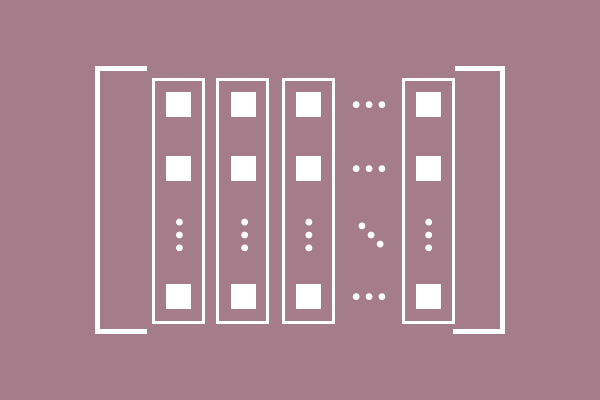# Column of a Matrix

A vertical line of elements in a matrix is called column of a matrix.The space inside a matrix is split as number of columns to place elements equally in each column. Every two elements in a column is separated by equal space.

Similarly, an equal space between every two columns is maintained in a matrix to avoid mixing elements of every two columns.

#### Example

$\begin{bmatrix} 1 & 0 & -3 & 2 \\ 4 & -7 & 7 & 5\\ -3 & 0 & 2 & -1\\ \end{bmatrix}$

1. The vertical line of elements $1,$ $4$ and $-3$ is called the first column of matrix.
2. The vertical line of elements $0,$ $-7$ and $0$ is called the second column of matrix.
3. The vertical line of elements $-3,$ $7$ and $2$ is called the third column of matrix.
4. The vertical line of elements $2,$ $5$ and $-1$ is called the fourth column of matrix.
Latest Math Topics
Jun 26, 2023
Jun 23, 2023

Latest Math Problems
Jul 01, 2023
Jun 25, 2023
###### Math Questions

The math problems with solutions to learn how to solve a problem.

Learn solutions

Practice now

###### Math Videos

The math videos tutorials with visual graphics to learn every concept.

Watch now

###### Subscribe us

Get the latest math updates from the Math Doubts by subscribing us.